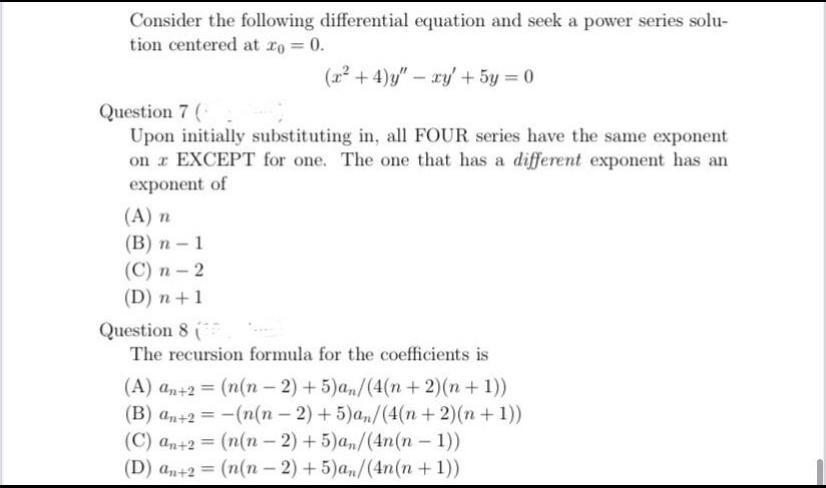Question:

# Consider the following differential equation and seek a power series solution centered at x₀ = 0. (x² + 4)y'' - xy' + 5y = 0 (7) Upon initially substituting in, all FOUR series have the same exponentConsider the following differential equation and seek a power series solution centered at x₀ = 0. (x² + 4)y'' - xy' + 5y = 0 (7) Upon initially substituting in, all FOUR series have the same exponent on x EXCEPT for one. The one that has a different exponent has an exponent of (A) n (B) n-1 (C) n - 2 (D) n+1 (8) The recursion formula for the coefficients is (A) an+2 = (n(n-2)+5)an/(4(n + 2)(n + 1)) (B) an+2 = -(n(n - 2)+5)an/(4(n + 2)(n+1)) (C) an+2 = (n(n-2) +5)an/(4n(n-1)) (D) an+2 = (n(n - 2) + 5)an /(4n(n + 1))